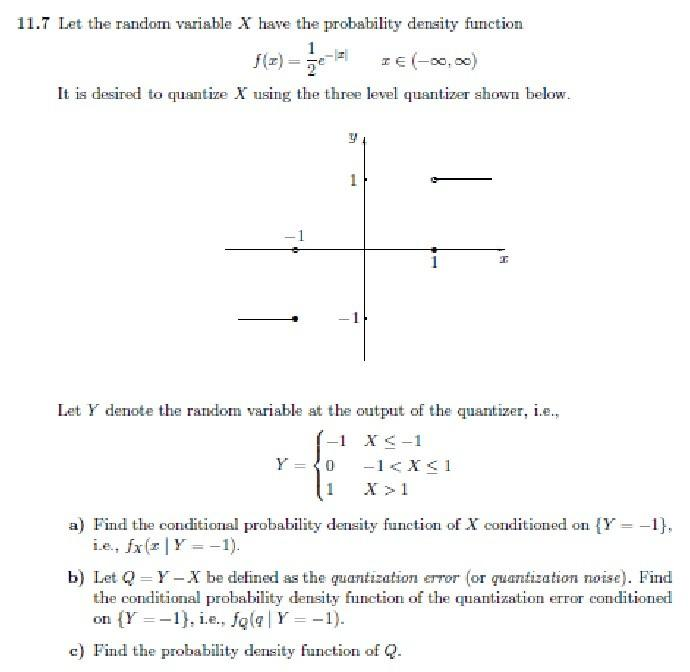Home / Expert Answers / Statistics and Probability / 1-7-let-the-random-variable-x-have-the-probability-density-function-f-x-21exx-pa247

# (Solved): 1.7 Let the random variable X have the probability density function f(x)=21exx( ...1.7 Let the random variable have the probability density function It is desired to quantize using the three level quantizer shown below. Let denote the random variable at the output of the quantizer, i.e., a) Find the conditional probability density function of conditioned on , i.e., . b) Let be defined as the quantization error (or quantization noise). Find the conditional probability density function of the quantization error conditioned on , i.e., . c) Find the probability density function of .

We have an Answer from Expert